# Android Apps for Science and Engineering

## Mathematics Apps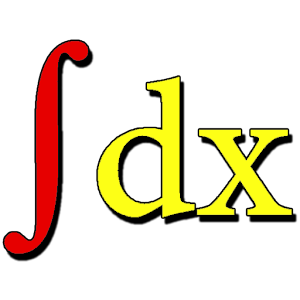Calculus Reference Price: FREE Contains formulas for derivatives, limits, series and integrals.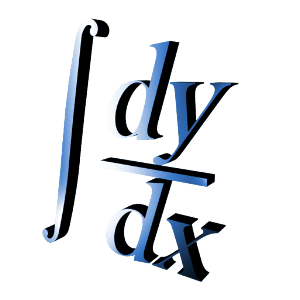Calculus Tools Price: FREE Math application that finds derivatives, computes definite integrals, plots graphs of functions, and more.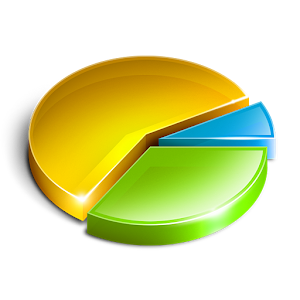Statistics Quick Reference Price: FREE Provides definitions, formulas, concepts in probability and statistics.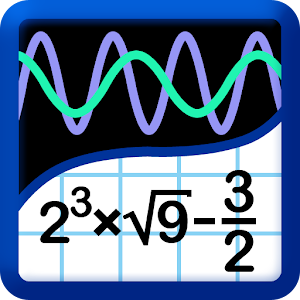Graphing Calculator (MathLab) Price: FREE Graphing calculator with algebra.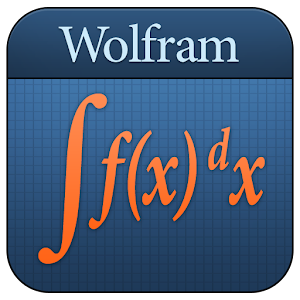Calculus Course Assistant Price: \$4.05 CAD This definitive app for calculus--from the world leader in math software--will help you work through your homework problems, ace your tests, and learn calculus concepts.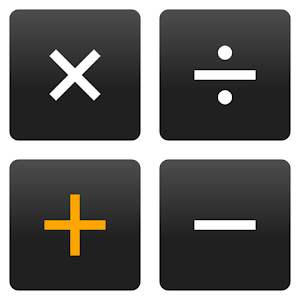RealCalc Plus Price: \$3.19 CAD A fully featured scientific calculator which looks and operates like the real thing.

## General Science Apps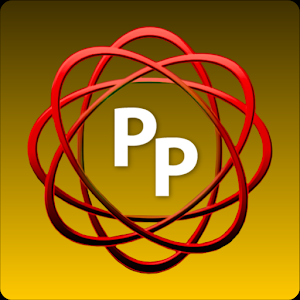Protocolpedia Price: FREE View lab methods and protocols - user account required for downloads.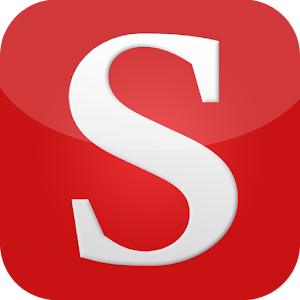Science Mobile Price: FREE Read summaries, abstracts, full-text articles (subscription required) from Science, Science Translational Medicine, and Science Signaling.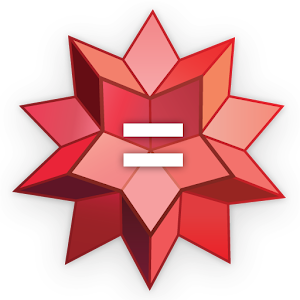Wolfram Alpha Price: \$2.95 CAD Across thousands of domains--with more continually added--Wolfram|Alpha uses its vast collection of algorithms and data to compute answers and generate reports for you.